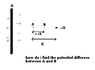# What i need to do in this situation

transgalactic
I unploaded a picture describing this situation

i don't know how to find the Potential difference in this situation
because there is two different objects. a charge and a plane.
on the plane we calculate the Potential difference
E*d=v1-v2
on the charge each potential calculates in V=Q/4pi*e0*r

to different things

problem description
there is an infinite plane whos charge densety is G

on a distance D we put a positve charge +Q

between this charge and the plane there is two point

A and B
point A is distant from the charge in X

point b is distant from the charge in 0.5X

how do i find the Potential difference
between these point?

#### Attachments

•p3.GIF
2.7 KB · Views: 494
Last edited: